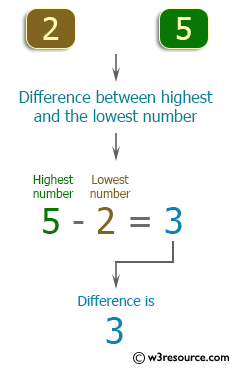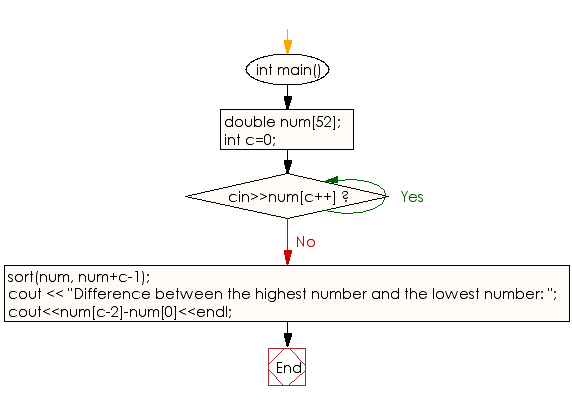﻿ C++ : Compute the difference between the highest and lowest

# C++ Exercises: Compute the difference between the highest number and the lowest number

## C++ Basic: Exercise-74 with Solution

Write a C++ program that accepts various numbers and computes the difference between the highest number and the lowest number. All input numbers should be real numbers between 0 and 1,000,000. The output (real numbers) may include an error of 0.01 or less.

Pictorial Presentation:Sample Solution:

C++ Code :

``````#include <iostream>
using namespace std;
int main()
{
double num;
int c=0;
while(cin>>num[c++]);
sort(num, num+c-1);
cout << "Difference between the highest number and the lowest number: ";
cout<<num[c-2]-num<<endl;
}
``````

Sample Output:

```Sample Input: 2 5
Difference between the highest number and the lowest number: 3
```

Flowchart:C++ Code Editor:

What is the difficulty level of this exercise?

﻿

## C++ Programming: Tips of the Day

Why is there no std::stou?

The most pat answer would be that the C library has no corresponding "strtou", and the C++11 string functions are all just thinly veiled wrappers around the C library functions: The std::sto* functions mirror strto*, and the std::to_string functions use sprintf.

Ref: https://bit.ly/3wtz2qA

We are closing our Disqus commenting system for some maintenanace issues. You may write to us at reach[at]yahoo[dot]com or visit us at Facebook